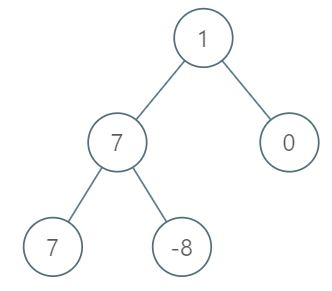# 最大层内元素和----leetcode周赛150_1002

### 示例 ：### 提示

1. 树中的节点数介于 1 和 10^4 之间
2. -10^5 <= node.val <= 10^5

### 思路：

• 利用队列，对二叉树进行层序遍历，记录每层节点和，逐步更新ans为最大和所在层数
• 可以利用变量，记录下一层节点的个数，每次利用for循环访问一层的节点，即可达到统计的目的

#### 具体代码如下 ：

class Solution {
public:
int ans =  0;int anshe = -2147483648;//ans层数与ans层和
int cenghe; int curceng;
queue<TreeNode*> que;
int maxLevelSum(TreeNode* root) {
if(!root)return 0;
int len =1;int next = 0;curceng = 0;//当前层个数，下一层节点个数，当前在第几层。
que.push(root);
while(que.size()){
cenghe = 0;//当前层的和
curceng ++;//当前在第几层
next = 0;//下一层个数
for(int i  = 0 ; i < len;i++){
TreeNode* t = que.front();que.pop();
cenghe += t->val;
if(t->left){que.push(t->left);next++;}
if(t->right){que.push(t->right);next++;}
}
len = next;
if(cenghe > anshe){
ans = curceng;
anshe = cenghe;
}
}
return ans;
}
};
posted @ 2019-08-18 16:11 我不是张小毛 阅读(...) 评论(...) 编辑 收藏